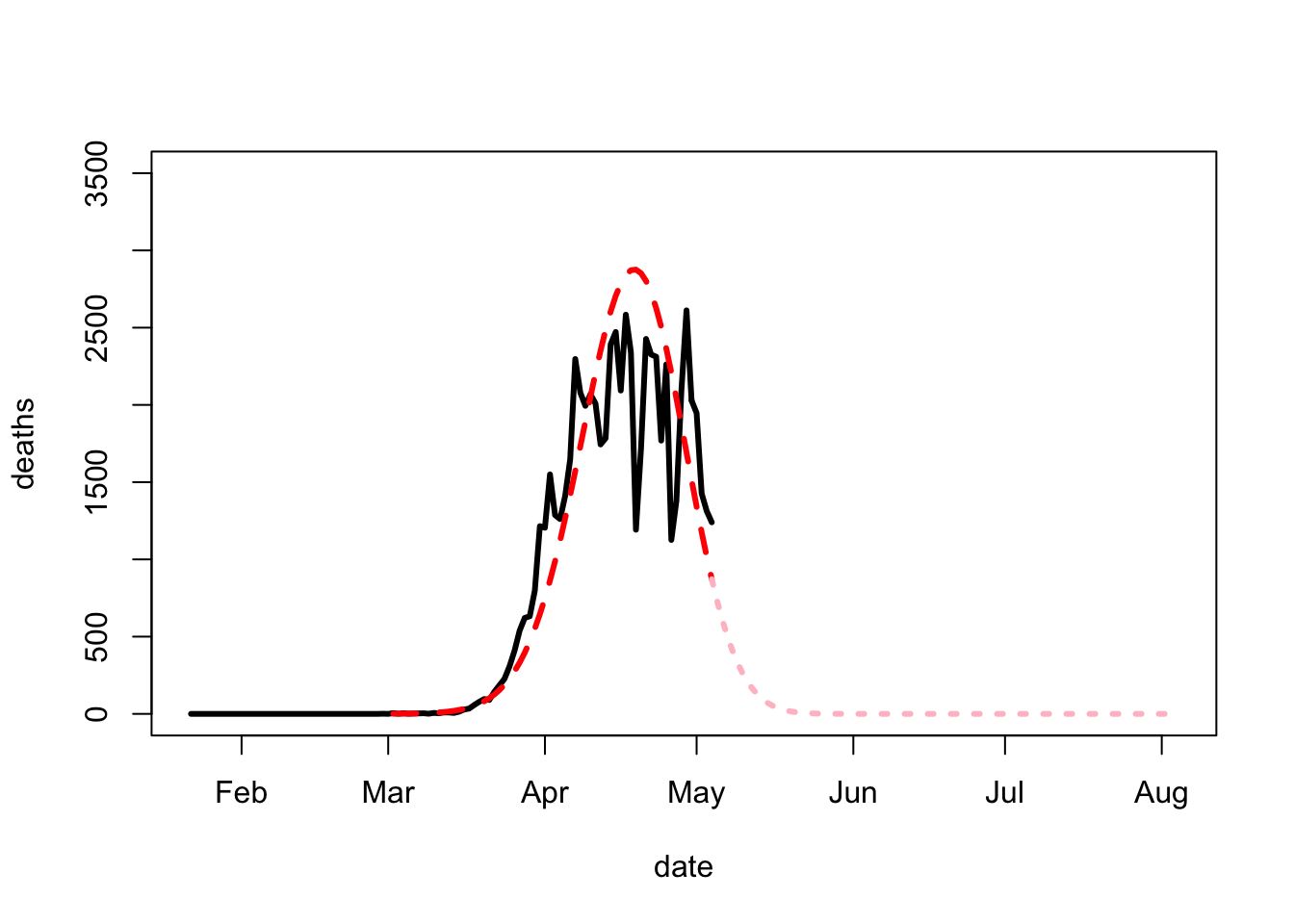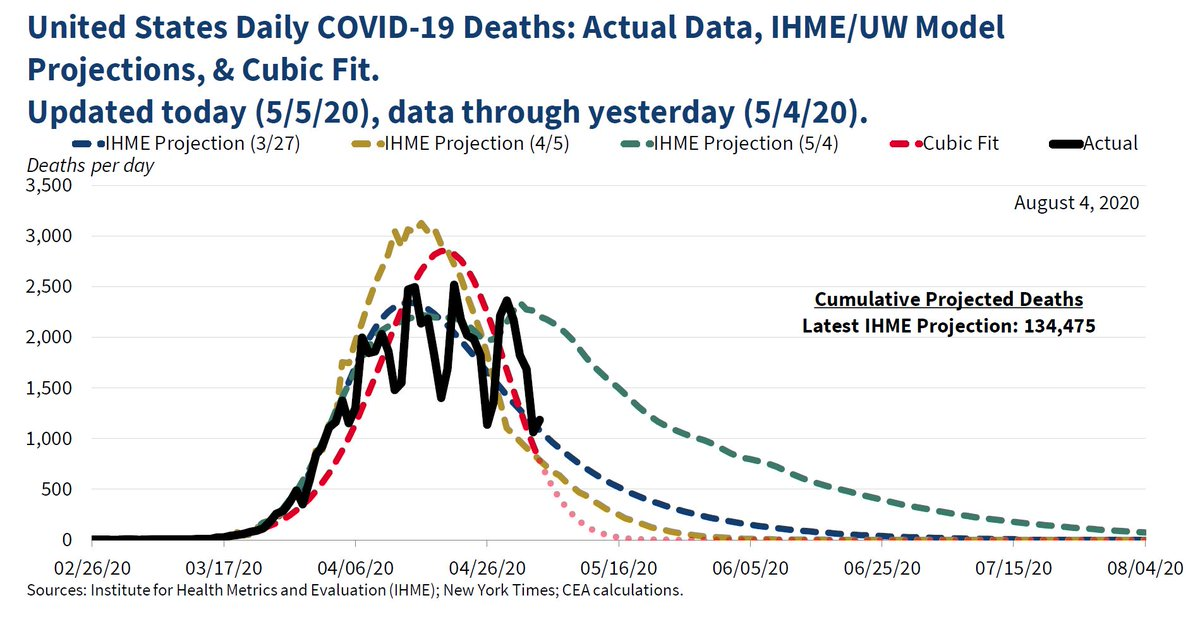## May 05, 2020

### How Not to Fit a Trend

If one of The Kids in Data Over Space and Time turned in something like this, I'd fail them ask where I'd gone wrong patiently talk them through all the reasons why blind, idiot curve-fitting is, in fact, idiotic, especially for extrapolating into the future. If they told me "well, I fit a cubic polynomial to the log of the series", we would go over why that is, still, blind, idiot curve-fitting.

library("covid19.analytics")
temp <- covid19.data("ts-deaths-US")
cu_deaths <- colSums(temp[,-(1:4)])
deaths <- c(0,diff(cu_deaths))
covid <- data.frame(cu_deaths,
deaths,
date=as.Date(names(cu_deaths)))
rownames(deaths) <- c()
plot(deaths ~ date, data=covid, type="l",
lty="solid",
ylim=c(0,3500),
xlim=c(min(covid$date), as.Date("2020-08-04")), lwd=3) start.date <- "2020-03-01" working.data <- covid[covid$date>start.date,]
cubic <- lm(log(deaths) ~ poly(date, 3),
data=working.data)
lines(x=working.data$date, y=exp(fitted(cubic)), col="red", lty="dashed", lwd=3) future.dates <- seq(from=max(working.data$date),
to=as.Date("2020-08-04"),
by=1)
lines(x=future.dates,
y=exp(predict(cubic,
newdata=data.frame(date=future.dates))),
lty="dotted", col="pink", lwd=3)Of course, by reinforcing one of the most basic lessons about time series, I'd evidently be depriving them of the chance to join the Council of Economic Advisors to the President of the United States:(Source)

I had always imagined that if we fell to pieces, it would be because we did something clever but deeply unwise. It is very depressing to realize that we may well end ourselves through sheer incompetence.

Posted at May 05, 2020 18:26 | permanent link